Chapter 4

As we know, the Gauss Law of Electric Field defines the relationship between electric charge and electric field as follows:(4.1)

In which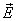is the electric field strength, and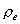is the electric charge density.

If we combine the Gauss Law of Electric Field equation (4.1) and the electric charge density distribution equation (2.1), we will obtain the follows electric field solution: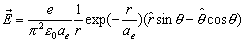(4.2)

Below, we will prove that the above equation (4.2) satisfies the Gauss Law of Electric Field.

As we know the gradient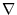in spherical coordinate is:From equation (4.2), thus: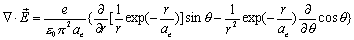(4.3)

Thus: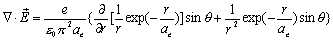(4.4)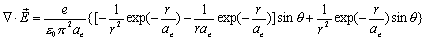(4.5)

Therefore:(4.6)

And so: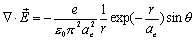(4.7)

If we combine equation (4.7) and (2.1), then we will get the Gauss Law of Electric Field.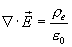Thus we have proved that the electric field equation (4.2) indeed satisfies the Gauss Law of Electric Field.